# 4. Fuzzy inference systems

A fuzzy inference system (FIS) is a system that uses fuzzy set theory to map inputs (features in the case of fuzzy classification) to outputs (classes in the case of fuzzy classification). Two FIS’s will be discussed here, the Mamdani and the Sugeno.

## 4.1 Fuzzy inference systems (Mamdani)

An example of a Mamdani inference system is shown in Figure 4-1. To compute the output of this FIS given the inputs, one must go through six steps:

1. determining a set of fuzzy rules

2. fuzzifying the inputs using the input membership functions,

3. combining the fuzzified inputs according to the fuzzy rules to establish a rule strength,

4. finding the consequence of the rule by combining the rule strength and the output membership function,

5. combining the consequences to get an output distribution, and

6. defuzzifying the output distribution (this step is only if a crisp output (class) is needed).

The following is a more detailed description of this process.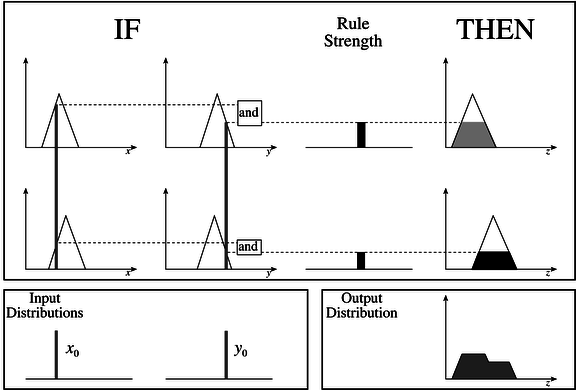Figure 4-1: A two input, two rule Mamdani FIS with crisp inputs

4.1.1 Creating fuzzy rules

Fuzzy rules are a collection of linguistic statements that describe how the FIS should make a decision regarding classifying an input or controlling an output. Fuzzy rules are always written in the following form:

if (input1 is membership function1) and/or (input2 is membership function2) and/or …. then (outputn is output membership functionn).

For example, one could make up a rule that says:

if temperature is high and humidity is high then room is hot.

There would have to be membership functions that define what we mean by high temperature (input1), high humidity (input2) and a hot room (output1). This process of taking an input such as temperature and processing it through a membership function to determine what we mean by "high" temperature is called fuzzification and is discussed in section 4.1.2. Also, we must define what we mean by "and" / "or" in the fuzzy rule. This is called fuzzy combination and is discussed in section 4.1.3.

4.1.2 Fuzzification

The purpose of fuzzification is to map the inputs from a set of sensors (or features of those sensors such as amplitude or spectrum) to values from 0 to 1 using a set of input membership functions. In the example shown in Figure 4-1, their are two inputs, x0 and y0 shown at the lower left corner. These inputs are mapped into fuzzy numbers by drawing a line up from the inputs to the input membership functions above and marking the intersection point.

These input membership functions, as discussed previously, can represent fuzzy concepts such as "large" or "small", "old" or "young", "hot" or "cold", etc. For example, x0 could be the EMG energy coming from the front of the forearm and y0 could be the EMG energy coming from the back of the forearm. The membership functions could then represent "large" amounts of tension coming from a muscle or "small" amounts of tension. When choosing the input membership functions, the definition of what we mean by "large" and "small" may be different for each input.

4.1.3 Fuzzy combinations (T-norms)

In making a fuzzy rule, we use the concept of "and", "or", and sometimes "not". The sections below describe the most common definitions of these "fuzzy combination" operators. Fuzzy combinations are also referred to as "T-norms".

4.1.3.1 Fuzzy "and"

The fuzzy "and" is written as: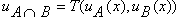where µA is read as "the membership in class A" and µB is read as "the membership in class B". There are many ways to compute "and". The two most common are:

1. Zadeh - min(uA(x), uB(x)) This technique, named after the inventor of fuzzy set theory simply computes the "and" by taking the minimum of the two (or more) membership values. This is the most common definition of the fuzzy "and".

2. Product - ua(x) times ub(x)) This techniques computes the fuzzy "and" by multiplying the two membership values.

Both techniques have the following two properties:

T(0,0) = T(a,0) = T(0,a) = 0

T(a,1) = T(1,a) = a

One of the nice things about both definitions is that they also can be used to compute the Boolean "and". Table 1 shows the Boolean "and" operation. Notice that both fuzzy "and" definitions also work for these numbers. The fuzzy "and" is an extension of the Boolean "and" to numbers that are not just 0 or 1, but between 0 and 1.

 Input1 (A) Input2 (B) Output (A "and" B) 0 0 0 0 1 0 1 0 0 1 1 1

Table 1: The Boolean "and"

4.1.3.2 Fuzzy "or"

The fuzzy "or" is written as: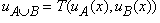Similar to the fuzzy "and", there are two techniques for computing the fuzzy "or":

1. Zadeh - max(uA(x), uB(x)) This technique computes the fuzzy "or" by taking the maximum of the two (or more) membership values. This is the most common method of computing the fuzzy "or".

2. Product - uA(x)+ uB(x) - uA(x) uB(x) This technique uses the difference between the sum of the two (or more) membership values and the product of the membership values.

Both techniques have the following properties:

T(a,0) = T(0,a) = a

T(a,1) = T(1,a) = 1

Similar to the fuzzy "and", both definitions of the fuzzy "or" also can be used to compute the Boolean "or". Table 2 shows the Boolean "or" operation. Notice that both fuzzy "or" definitions also work for these numbers. The fuzzy "or" is an extension of the Boolean "or" to numbers that are not just 0 or 1, but between 0 and 1.

 Input1 (A) Input2 (B) Output (A "or" B) 0 0 0 0 1 1 1 0 1 1 1 1

Table 2: The Boolean "or"

4.1.4 Consequence

The consequence of a fuzzy rule is computed using two steps:

1. Computing the rule strength by combining the fuzzified inputs using the fuzzy combination process discussed in section 4.1.3. This is shown in Figure 4-1. Notice in this example, the fuzzy "and" is used to combine the membership functions to compute the rule strength.

2. Clipping the output membership function at the rule strength. Once again, refer to Figure 4-1 to see how this is done for a two input, two rule Mamdani FIS.

4.1.5 Combining Outputs into an Output Distribution

The outputs of all of the fuzzy rules must now be combined to obtain one fuzzy output distribution. This is usually, but not always, done by using the fuzzy "or". Figure 4-1 shows an example of this. The output membership functions on the right hand side of the figure are combined using the fuzzy "or" to obtain the output distribution shown on the lower right corner of the figure.

4.1.6 Defuzzification of Output Distribution

In many instances, it is desired to come up with a single crisp output from a FIS. For example, if one was trying to classify a letter drawn by hand on a drawing tablet, ultimately the FIS would have to come up with a crisp number to tell the computer which letter was drawn. This crisp number is obtained in a process known as defuzzification. There are two common techniques for defuzzifying:

1. Center of mass - This technique takes the output distribution found in section 4.1.5 and finds its center of mass to come up with one crisp number. This is computed as follows: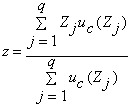where z is the center of mass and uc is the membership in class c at value zj. An example outcome of this computation is shown in Figure 4-2.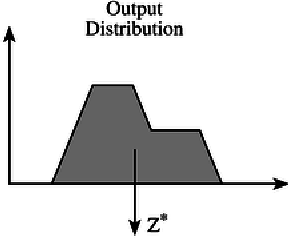Figure 4-2: Defuzzification Using the Center of Mass

2. Mean of maximum - This technique takes the output distribution found in section 4.1.5 and finds its mean of maxima to come up with one crisp number. This is computed as follows: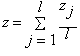where z is the mean of maximum, zj is the point at which the membership function is maximum, and l is the number of times the output distribution reaches the maximum level. An example outcome of this computation is shown in Figure 4-3.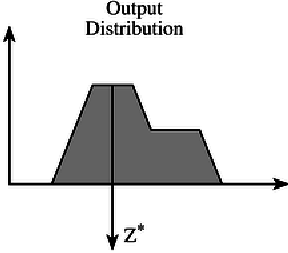Figure 4-3: Defuzzification Using the Mean of Maximum

4.1.7 Fuzzy Inputs

In summary, Figure 4-1 shows a two input Mamdani FIS with two rules. It fuzzifies the two inputs by finding the intersection of the crisp input value with the input membership function. It uses the minimum operator to compute the fuzzy "and" for combining the two fuzzified inputs to obtain a rule strength. It clips the output membership function at the rule strength. Finally, it uses the maximum operator to compute the fuzzy "or" for combining the outputs of the two rules.

Figure 4-4 shows a modification of the Mamdani FIS where the input y0 is fuzzy, not crisp. This can be used to model inaccuracies in the measurement. For example, we may be measuring the output of a pressure sensor. Even with the exact same pressure applied, the sensor is measured to have slightly different voltages. The fuzzy input membership function models this uncertainty. The input fuzzy function is combined with the rule input membership function by using the fuzzy "and" as shown in Figure 4-4.Figure 4-4: A two Input, two rule Mamdani FIS with a fuzzy input

## 4.2 Fuzzy Inference System (Sugeno)

The Sugeno FIS is quite similar to the Mamdani FIS discussed in section 4. The primary difference is that the output consequence is not computed by clipping an output membership function at the rule strength. In fact, in the Sugeno FIS there is no output membership function at all. Instead the output is a crisp number computed by multiplying each input by a constant and then adding up the results. This is shown in Figure 4-5. "Rule strength" in this example is referred to as "degree of applicability" and the output is referred to as the "action". Also notice that there is no output distribution, only a "resulting action" which is the mathematical combination of the rule strengths (degree of applicability) and the outputs (actions).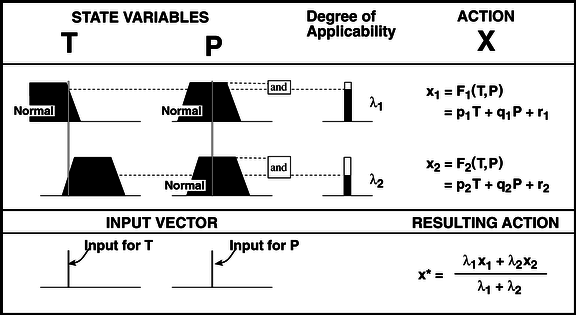Figure 4-5: A two input, two rule Sugeno FIS (pn, qn, and rn are user-defined constants)

One of the large problems with the Sugeno FIS is that there is no good intuitive method for determining the coefficients, p, q, and r. Also, the Sugeno has only crisp outputs which may not be what is desired in a given HCI application. Why then would you use a Sugeno FIS rather than a Mamdani FIS? The reason is that there are algorithms which can be used to automatically optimize the Sugeno FIS. One of these algorithms is discussed in section 5.1.2

In classification, p and q can be chosen to be 0 and r can be chosen to be a number that corresponds to a particular class. For example, if we wanted to use the EMG from a person’s forearm to classify which way his/her wrist was bending, we could assign the class "bend_inward" to have the value r = 1. We could assign the class "bend_outward" to have the value r=0. Finally, we could assign the class "no_bend" to have the value r=0.5.